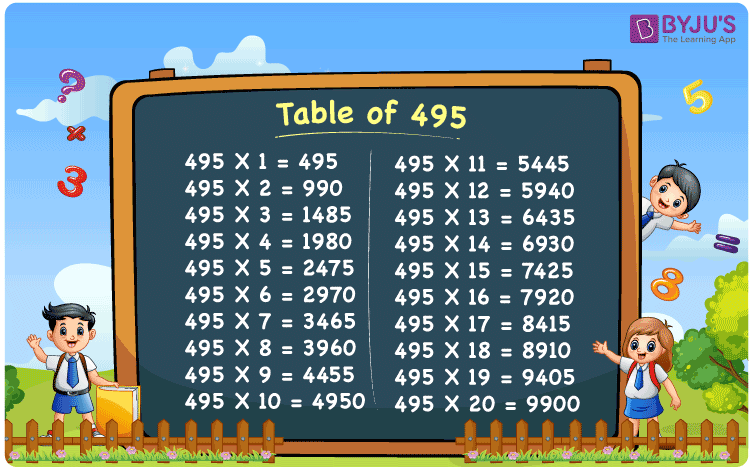Checkout JEE MAINS 2022 Question Paper Analysis : Checkout JEE MAINS 2022 Question Paper Analysis :

# Table of 495

The Table of 495 is very essential in Mathematics for students who aspire to score good marks in their exams. Multiplication, addition, subtraction and division are some of the basic concepts. If a student masters these concepts, performing well in the annual exams will be very easy. Using the multiplication table of 495 provided at BYJU’S, students will get a stronghold on the method of multiplying a particular number with all natural numbers.

## Table of 495 Chart## What is the 495 Times Table?

Students need to focus on the multiplication table of 495 from their primary education so that the fundamental topics can be learnt thoroughly. Students can find below how a number 495 is multiplied with natural numbers till 10. Multiplication is a process of adding a particular number with the number of times it should be multiplied.

 495 × 1 = 495 495 495 × 2 = 990 495 + 495 = 990 495 × 3 = 1485 495 + 495 + 495 = 1485 495 × 4 = 1980 495 + 495 + 495 + 495 = 1980 495 × 5 = 2475 495 + 495 + 495 + 495 + 495 = 2475 495 × 6 = 2970 495 + 495 + 495 + 495 + 495 + 495 = 2970 495 × 7 = 3465 495 + 495 + 495 + 495 + 495 + 495 + 495 = 3465 495 × 8 = 3960 495 + 495 + 495 + 495 + 495 + 495 + 495 + 495 = 3960 495 × 9 = 4455 495 + 495 + 495 + 495 + 495 + 495 + 495 + 495 + 495 = 4455 495 × 10 = 4950 495 + 495 + 495 + 495 + 495 + 495 + 495 + 495 + 495 + 495 = 4950

## Multiplication Table of 495

The Table of 495 is provided in the form of charts, solved examples and PDF which can be accessed by the students to get their doubts clarified instantly. The main objective of preparing the table is to provide students with the best reference material while learning new concepts. During the competitive exams, having a thorough knowledge of the multiplication tables will help in saving a lot of time.

 495 × 1 = 495 495 × 2 = 990 495 × 3 = 1485 495 × 4 = 1980 495 × 5 = 2475 495 × 6 = 2970 495 × 7 = 3465 495 × 8 = 3960 495 × 9 = 4455 495 × 10 = 4950 495 × 11 = 5445 495 × 12 = 5940 495 × 13 = 6435 495 × 14 = 6930 495 × 15 = 7425 495 × 16 = 7920 495 × 17 = 8415 495 × 18 = 8910 495 × 19 = 9405 495 × 20 = 9900

## Solved Example on the Table of 495

Q.1: At the shelter, 18 dogs were adopted in a day. How many dogs will be adopted in 495 days?

Solution: Given,

Number of dogs that were adopted in a day = 18

In 495 days number of dogs that will be adopted = 495 x 18

= 8910

## Frequently Asked Questions on the Table of 495

### Find the value of 495 multiplied by 16.

From the table of 495,
495 multiplied by 16 is 495 x 16 = 7920.

### Determine the value which should be multiplied with 495 to get 5940.

In the table of 495,
495 x 12 = 5940.
Hence, the value which should be multiplied with 495 to get 5940 is 12.

### What is 495 times 13 plus 9 minus 3?

With the help of the multiplication table of 495,
495 times 13 plus 9 minus 2 can be written as
495 x (13 + 9 – 3)
= 495 x 19
= 9405